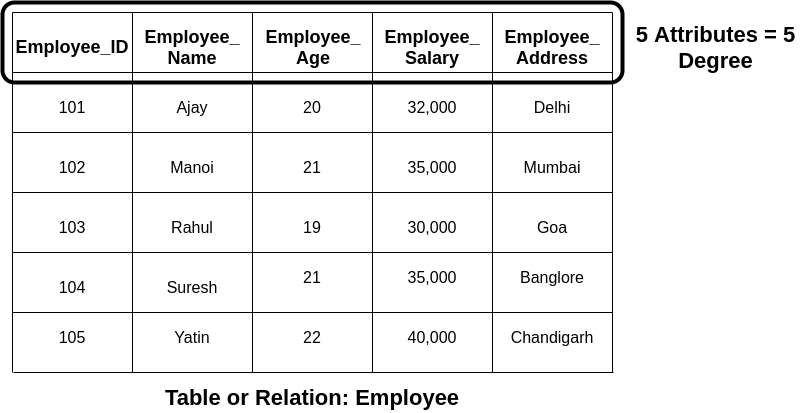Relational Model in DBMS - MetaTutorials
Here, we will study about the Relational Model and its basic concepts in detail with examples. Here, we will also learn about the advantages and disadvantages of the Relational Model.

The Computer Scientist Edgar Frank Codd (E.F. Codd) proposed the Relational Model in 1969 for the database management system. It is used widely in the entire world for data storing and processing.
When the ER diagram of the database is completely designed, then the RDBMS languages convert the ER diagram into the Relational model.

## What is Relational Model?

A relational model is a model which represents the inserted data or information in the form of tables. In relational databases, tables are known as relations. The tables contain rows and columns which are called Tuples and Attributes in the relational database.This model is called relational because the data in each table of the database are related to each other.
Following are the various popular Relational Database Management system which used for managing the data in relational databases:
• Oracle,
• DB2,
• Microsoft SQL Server,
• Microsoft Access,
• SQLite, etc.

## Characteristics of Relational Model

Following are the various features or characteristics of the Relational Model:
• The relational model represents the data in the table, which is composed of rows and columns.
• Users can easily access and share the data in the relational database model.
• The relational model provides data consistency and accuracy.
• The relational model provides a facility of a primary key, which uniquely identifies each record or row of the tables.

## Table

A table stores all the data inserted by the user in the relational database. In the tables, data is stored in the cell, which is a combination of row and column. In the database, each table has a particular name and is designed for a particular purpose.
Rows indicate the records and the columns indicate the attributes of a relation (table).
The following diagram shows the Employee table (relation), which has 5 rows and 5 columns.

## Attribute

An attribute is the column of the table. Attributes are the characteristics or properties which define the relation. In the relational database, an attribute is also known as a field. A table has a specific number of attributes.
The following table has Five attributes whose names are:
1. Employee_ID
2. Employee_Name
3. Employee_Age
4. Employee_Salary

## Record

Record is a single row of data in the relational table. The record is also known as a tuple.
The following Employee table contains 5 records/rows/tuples:

## Relation Schema

In the relational model, a relation schema denotes the name of a relation with its properties (attributes or fields). It describes the structure of the relation.
For an Employee relation, the relation schema is like this:
EMPLOYEE (EMPLOYEE_ID, EMPLOYEE_NAME, EMPLOYEE_AGE, EMPLOYEE_SALARY, EMPLOYEE_ADDRESS)

## Relation Key

In the relational database, a relation key is a field that uniquely denotes the specific row of the table.
In the following table, Employee_ID is the relational key.

## Relation Instance

In the relational database, the relation instance is the finite set of records/rows/tuples present in the relation at a specific moment. Data duplicacy is not allowed in this concept.

## Degree

In the relational database, the degree is the total number of fields or attributes present in the relation.
The following table employee has degree 5.## Cardinality

In the relational database, cardinality is the total number of records or rows present in the table.
The following table Employee has cardinality .

## Domain

In the relational database, every attribute of relation holds only a certain type of value, which is known as the domain of attribute.

Following are the advantages or benefits of Relational Model:
• This model is so simple than the network and hierarchical model.
• The relational model consists of tables, so it is easy to use and understand.
• It provides better data security and integrity.
• This model hides the details about the physical storage from the database users and developers.
• Data accuracy is more in the relational model than the other data models. Because, the tables are related to each other in the relational databases, that's why there is no chance for data duplicacy.
• This model also provides procedures for data backup.